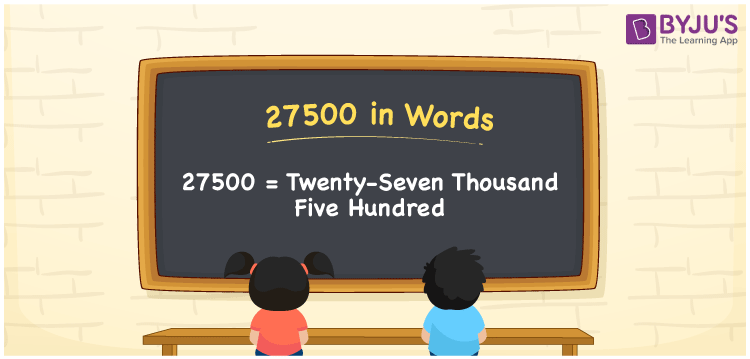# 27500 in Words

We can express 27500 in words as Twenty-Seven Thousand Five Hundred. If you paid Rs. 27500 to buy a new mobile, you can say, “I bought a new mobile worth Twenty-Seven Thousand Five Hundred rupees”. We know that 27500 is a cardinal number since it denotes a certain amount. In this article, students will learn how to express 27500 in words.

 27500 in words Twenty-Seven Thousand Five Hundred Twenty-Seven Thousand Five Hundred in numerical form 27500

## 27500 in English Words

We know that numbers in words are written using the English alphabet. Thus, to write numbers in words students are advised to learn the English language, as it is the most commonly used language in the education field. Hence, 27500 in words is written as Twenty-Seven Thousand Five Hundred.## How to Write 27500 in Words?

27500 can be converted using a place value chart. Since 27500 has 5 digits. So, let’s make a chart that has a place value of up to 5 digits.

 Ten-Thousands Thousands Hundreds Tens Ones 2 7 5 0 0

Therefore, we can write the expanded form as:

2 x Ten-Thousand + 7 x Thousand + 5 x Hundred + 0 × Ten + 0 × One

= 2 x 10000 + 7 x 1000 + 5 x 100 + 0 + 0

= 20000 + 7000 + 500

= 27500

= Twenty-Seven Thousand Five Hundred

Hence, 27500 in words is written as Twenty-Seven Thousand Five Hundred.

Interesting way of writing 27500 in words

2 = Two

27 = Twenty-Seven

275 = Two Hundred and Seventy-Five

2750 = Two Thousand Seven Fifty

27500 = Twenty-Seven Thousand Five Hundred

Thus, the word form of the number 27500 is Twenty-Seven Thousand Five Hundred.

27500 is a natural number that is succeeded by 27499 and preceded by 27501

• 27500 in words – Twenty-Seven Thousand Five Hundred
• Is 27500 an odd number? – No
• Is 27500 an even number? – Yes
• Is 27500 a perfect square number? – No
• Is 27500 a perfect cube number? – No
• Is 27500 a prime number? – No
• Is 27500 a composite number? – Yes

## Frequently Asked Questions on 27500 in Words

Q1

### How do you write 27500 in words?

27500 in words is written as Twenty-Seven Thousand Five Hundred.
Q2

### Write the value of 20000 + 7500 in words.

20000 + 7500 = 27500 Therefore, the sum of Twenty Thousand and Seven Thousand Five Hundred is Twenty-Seven Thousand Five Hundred.
Q3

### 27500 is a composite number. True or False.

True, 27500 is a composite number.